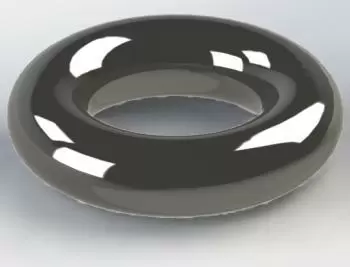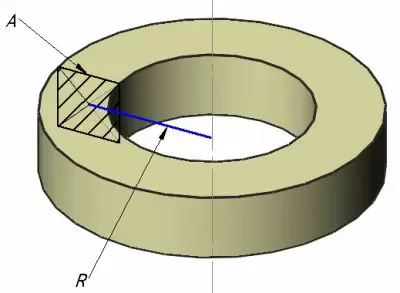# What Is a Toroid in Geometry? Definition, Surface, and VolumeA toroid is a solid shape generated by rotating a plane geometric shape around an axis outside the shape's area. A toroid is a type of solid of revolution with the apperence of a hollow circular ring or a doughnut-shaped solid.

A surface of revolution is created by revolving a plane curve about a straight line that lies in the same plane, the axis of rotation. In the case of a toroid, the plane curve must be a closed shape, and the axis of rotation cannot intersect the perimeter.

This type of three-dimensional solids is also known by the name of annular surface.

Toroids are frequently used in the field of electricity. For example, passing an electrical current through an electrical cable wound in a toroidal shape creates a field generated by a magnetic flux. This magnetic field is used in applications such as electric motors.

## What Is a Torus?

A torus is a particular case of a toroid in which the geometric shape in rotation and the trajectory are circles. Thus, a torus is a surface of revolution obtained by rotating the generating circle around an axis that lies in the plane of this circle and does not intersect it.

## Characteristics of a Toroid: Surface and Volume

We can specify a torus by the radius of the circle of revolution R, the distance from the center of the rotated shape to the axis of revolution.

If the surfaces of revolution of a toroid are symmetric, we can calculate the surface and the volume.

### Calculate the Area and Volume of a Square ToroidThe following formulas are used to find the volume (V) and the surface area (S) of a toroid with a square section, and R is the radius of revolution.

V= 2πRA

S = 2πRP

Where:

• R is the radius of revolution (from the center of the square to the axis of rotation)

• A is the area of ​​the cross-section.

• P is the perimeter of the square.

### Calculate the Area and Volume of a Torus

To calculate the volume (V) and the surface area (S) of a circular toroid with a circumference of radius r, we will use the following formulas:

are given by the following equations, where r is the radius of the circular section and R is the radius of the general shape.

V=2π2r2R

S=4π2rR

Where:

• R is the radius of revolution (from the center of the circle to the axis of rotation)

• r is the radius of the circumference.

Author:

Published: February 20, 2022
Last review: February 20, 2022